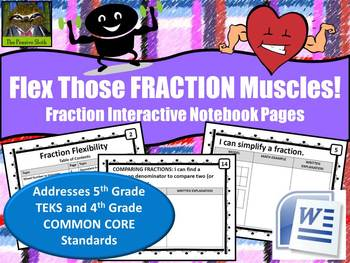# Fraction Flexibility--Interactive Math Notebook Pages (TEKS & COMMON CORE)**WORDSubject
Resource Type
File Type
Word Document File (786 KB|16 slides, 2 per page, 8 pages)
\$1.00
Product Description
There are two versions of this product so that you can download it in the format that best meets your needs. They are both the same price.

*Click HERE for the zipped file that contains both the printer-friendly PDF version, editable PowerPoint version, and a Microsoft Word booklet version with two slides tiled on a page to save paper.

*Click HERE for the product as an easy to print Microsoft Word booklet with two slides tiled on a page to save paper.

INTRODUCTION
The purpose of this interactive notebook set is for students to secure strategies for working with fractions by recording their own examples and writing critically about them. Before students can be successful performing operations with fractions, they have to be able to think flexibly about them. For example, they must know that 3/5 can also be 9/15, is equivalent to .60, is greater than 1/2, and much more!

Students find working with fractions much easier when they can see a fraction as a value that can be represented in a variety of ways AND when they can manipulate a fraction (keeping the value the same) so that it is easy to work with in each situation.

DESCRIPTION
*Product contains 16 interactive math notebook pages to be printed and made into a booklet to add to students' math journals

*Each page contains an "I Can..." statement with the skill, such as "I can simplify a fraction." Students then include an example showing the steps mathematically and write an explanation or how-to.

*"I CAN..." statements include:
--Improper fractions and mixed numbers
--Simplifying fractions
--Equivalent fractions
--Turning fractions into decimals when the denominator EASILY multiplies to 100
--Turning fractions into decimals when the denominator DOES NOT easily multiply to 100
--Comparing fractions by manipulating the denominator
--Comparing fractions by converting to decimals
--Comparing fractions by placing them on a 0, 1/2, 1 number line

*This product download contains a Microsoft Word doc with 2 slides tiled per page to save paper and print as a booklet! If you are interested in getting this product in a zipped file that contains the Word doc, PDF of each slide, and editable PowerPoint, please visit my TPT store.

HOW TO USE THIS PRODUCT
*After you have taught each skill, have students record a sample problem and write about it--critical writing improves learning!

*Print as a booklet and have students tape or glue the booklet into their math notebooks/journals so that they can refer to it later if they need a refresher

*This product is not a set of worksheets with problems for students to complete

DISCLAIMER
As a Texas teacher, the products I make are designed around the standards for the state of Texas, known as the Texas Essential Knowledge and Skills (TEKS) and are carefully crafted to help students be successful on the Texas STAAR test and other high-stakes tests. I use the rigor of the TEKS and released STAAR assessments as models and guides when putting together lessons and materials for my students. Thus, I am careful to post products that, in my judgment, align with these standards. I am not affiliated in any way with these entities and any and all claims of alignment are my claims, not those of the groups behind the TEKS or STAAR test.

There are many teachers in Common Core states looking for quality materials. If one of my TEKS based products addresses standards in the Common Core, I will include those standards in this description. I do not teach the Common Core in my classroom, but am familiar with the standards. I am not affiliated with the entity behind Common Core and all claims of alignment are my judgment, not those of the groups behind the Common Core.

***************TEKS***************
(5.2) Number, operation, and quantitative reasoning. The student uses fractions in problem-solving situations. The student is expected to:

(A) generate a fraction equivalent to a given fraction such as 1/2 and 3/6 or 4/12 and 1/3;

(B) generate a mixed number equivalent to a given improper fraction or generate an improper fraction equivalent to a given mixed number;

(C) compare two fractional quantities in problem-solving situations using a variety of methods, including common denominators; and

(D) use models to relate decimals to fractions that name tenths, hundredths, and thousandths.

***************COMMON CORE ***************
CCSS.Math.Content.4.NF.A.1 Explain why a fraction a/b is equivalent to a fraction (n × a)/(n × b) by using visual fraction models, with attention to how the number and size of the parts differ even though the two fractions themselves are the same size. Use this principle to recognize and generate equivalent fractions.

CCSS.Math.Content.4.NF.A.2 Compare two fractions with different numerators and different denominators, e.g., by creating common denominators or numerators, or by comparing to a benchmark fraction such as 1/2. Recognize that comparisons are valid only when the two fractions refer to the same whole. Record the results of comparisons with symbols >, =, or <, and justify the conclusions, e.g., by using a visual fraction model.

CCSS.Math.Content.4.NF.C.5 Express a fraction with denominator 10 as an equivalent fraction with denominator 100, and use this technique to add two fractions with respective denominators 10 and 100.2 For example, express 3/10 as 30/100, and add 3/10 + 4/100 = 34/100.

CCSS.Math.Content.4.NF.C.6 Use decimal notation for fractions with denominators 10 or 100. For example, rewrite 0.62 as 62/100; describe a length as 0.62 meters; locate 0.62 on a number line diagram.

CCSS.Math.Content.4.NF.C.7 Compare two decimals to hundredths by reasoning about their size. Recognize that comparisons are valid only when the two decimals refer to the same whole. Record the results of comparisons with the symbols >, =, or <, and justify the conclusions, e.g., by using a visual model.
Total Pages
16 slides, 2 per page, 8 pages